# RD Sharma Class 9 Solutions Chapter 6 Factorisation of Polynomials Ex 6.1

## RD Sharma Class 9 Solutions Chapter 6 Factorisation of Polynomials Ex 6.1

These Solutions are part of RD Sharma Class 9 Solutions. Here we have given RD Sharma Class 9 Solutions Chapter 6 Factorisation of Polynomials Ex 6.1

Other Exercises

Question 1.
Which of the following expressions are polynomials in one variable and which are not? State reasons for your answer: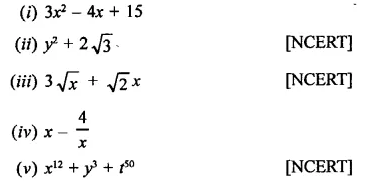Solution:
(i) 3x2 – 4x + 15,
(ii) y2 + 2$$\sqrt { 3 }$$ are polynomial is one variable. Others are not polynomial or polynomials in one variable.

Question 2.
Write the coefficient of x2 in each of the following: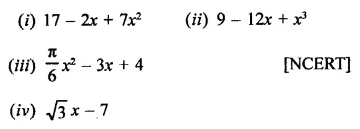Solution:
Coefficient of x2,
in (i) is 7
in (ii) is 0 as there is no term of x2 i.e. 0 x2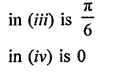Question 3.
Write the degrees of each of the following polynomials:
(i) 7x3 + 4x2 – 3x + 12
(ii) 12 – x + 2x3
(iii) 5y – $$\sqrt { 2 }$$
(iv) 7
(v) 0
Solution:
(i) Degree of the polynomial 7x3 + 4x2 – 3x + 12 is 3
(ii) Degree of the polynomial 12 – x + 2x3 is 3
(iii) Degree of the polynomial 5y – $$\sqrt { 2 }$$is 1
(iv) Degree of the polynomial 7 is 0
(v) Degree of the polynomial 0 is 0 undefined.

Question 4.
(i) x + x2 + 4
(ii) 3x – 2
(iii) 2x + x2 [NCERT]
(iv) 3y
(v) t2 + 1
(v) 7t4 + 4t3 + 3t – 2
Solution:
(i)  x + x2 + 4 It is a quadratic polynomial.
(ii) 3x – 2 : It is a linear polynomial.
(iii) 2x + x2: It is a quadratic polynomial.
(iv) 3y It is a linear polynomial.
(v) t2+ 1 It is a quadratic polynomial.
(vi) 7t4 + 4t3 + 3t – 2 It is a biquadratic polynomial.

Question 5.
Classify the following polynomials as polynomials in one-variable, two-variables etc.
(i) x2-xy +7y2
(ii) x2 – 2tx + 7t2 – x + t
(iii) t3 -3t2 + 4t-5
(iv) xy + yz + zx
Solution:
(i) x2 – xy + 7y2: It is a polynomial in two j variables x, y.
(ii) x2 – 2tx + 7t2 – x + t: It is a polynomial in two variables in x, t.
(iii) t3 – 3t2 + 4t – 5 : It is a polynomial in one variable in t.
(iv) xy +yz + zx : It is a polynomial in 3 variables in x, y and

Question 6.
Identify polynomials in the following: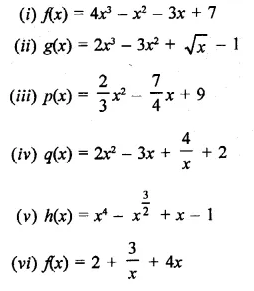Solution: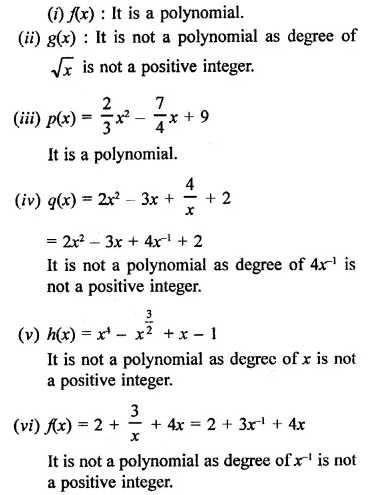Question 7.
Identify constant, linear, quadratic and cubic polynomials from the following polynomials: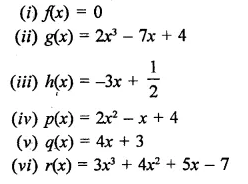Solution:
(i) f(x) = 0 : It is a constant polynomial as it has no variable.
(ii) g(x) = 2x3 – 7x + 4 : It is a cubic polynomial.
(iii) h(x) = -3x + $$\frac { 1 }{ 2 }$$ : It is a linear polynomial.
(iv) p(x) = 2x2 – x + 4 : It is a quadratic polynomial.
(v) q(x) = 4x + 3 : It is linear polynomial.
(vi) r(x) = 3x3 + 4x2 + 5x – 7 : It is a cubic polynomial.

Question 8.
Give one example each of a binomial of degree 35 and of a monomial of degree 100.   [NCERT]
Solution:
Example of a binomial of degree 35 = 9x35 + 16
Example of a monomial of degree 100 = 2y100

Hope given RD Sharma Class 9 Solutions Chapter 6 Factorisation of Polynomials Ex 6.1 are helpful to complete your math homework.

If you have any doubts, please comment below. Learn Insta try to provide online math tutoring for you.